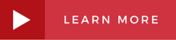# II-VI Marlow Blog

The transport properties of a solid includes the Seebeck coefficient (“S” in Volts per degree), the electrical conductivity (“s” in 1/(ohm-meters)) and the thermal conductivity (“l” in Watts per meter-Kelvin).  These three properties determine the thermoelectric figure of merit (Z) performance, Z º S2s/l, which has units of reciprocal temperature.### Thermoelectric Figure of Merit

From an engineering perspective, the Seebeck coefficient is defined as the ratio of electric potential difference to temperature difference in the absence of electrical current flow.  The Seebeck coefficient of a material can be measured using two thermocouples plus a heater, which establishes a small temperature difference across the material sample.  Accurate and precise measurement of a sample's temperature at two locations, typically a few millimeters apart, is the most challenging aspect of this measurement.

Electrical conductivity is defined as the ratio of electrical current density to electric field strength, in the absence of a temperature gradient.  In practice, electrical conductivity is obtained by measuring the voltage drop (V) across a sample of length (L) and constant cross-section (A) when a uniform current (I) is flowing: s = LI/AV.  For this measurement, it is important that the sample has a uniform current density and that the measured voltage does not contain any significant contact, or connector, resistances.

The thermal conductivity is defined as the ratio of heat flux to temperature gradient in the absence of electrical current flow.  Thermal conductivity can be obtained by measuring the temperature difference (DT) across a sample of length (L) and constant cross-section (A) when a heat flow (Q) is established: l = LQ/ADT.  Measurement errors in both heat flux and temperature make this a challenging property to quantify.

Many thermoelectric groups use a ‘4-probe’ method to collect S and s data concurrently on the same sample as a function of temperature.  In this measurement method, current is injected through the end faces of bar-shaped samples and thermocouples are pressed against a side face of the sample.  The resulting thermocouple-sample thermal contact may not necessarily be ideal and could possibly lead to an underestimation of temperature difference and consequent overestimation of S.  Errors in 4-probe Seebeck measurements have been studied recently (J. Martin, W. Wong-Ng & M. Green, Journal of Electronic Materials 44 #6, pp 1998-2006.) and found to be significant, especially at higher temperatures.

To obtain thermal conductivity, many researchers use an indirect method rather than the direct method mentioned above.  The indirect method requires measurement of the thermal diffusivity (cm2/sec, the ratio of the time derivative of temperature to the 2nd spatial derivative of temperature), the specific heat (Joules per gram-Kelvin) and the mass density (g/cm3).  Thermal diffusivity often is measured using a laser flash apparatus (http://www.electronics-cooling.com/2002/05/flash-diffusivity-method-a-survey-of-capabilities/), while specific heat is measured using a differential scanning calorimeter or differential thermal analyzer.

For the measurement methods most used by materials research groups interested in thermoelectric power generation, a recent empirical assessment of errors in the thermoelectric transport properties, led by a group at Oak Ridge National Laboratory, found the following reproducibility results for measurements from 323 to 773 Kelvin: ~±7% for S, ~±8% for s, ~±8% for l, ~±15% for Z.

For temperatures from 180 to 400 Kelvin, covering essentially all thermoelectric cooling applications, II-VI Marlow uses a modified Harman method to measure the thermoelectric properties.  This method uses the Peltier effect to create a temperature difference, so that no heater is required.  A switched DC current is used, and the Seebeck and ohmic voltage contributions are separated transiently, taking advantage of the low thermal diffusivity of thermoelectric materials.  Thermocouples are embedded in small copper blocks that are soldered to the material sample, which typically has a thin layer of nickel applied to the end faces.  Fine current and thermocouple wires are used to minimize thermal losses, which are estimated and used to make corrections to the calculated thermal conductivity.  II-VI Marlow believes the Z values obtained have an accuracy of ~±3%.

Whether you’re exploring outer space, working to develop the next cure for cancer or finding sustainable energy solutions, let us partner with you to help reach your goal. For specific information, contact II-VI Marlow and we will assist with the application and cooler selection